# 程式人雜誌 -- 2013 年 12 月號 (開放公益出版品)

## R 講題分享 - 種類數的估計與預測

### 研究問題與資料格式

i 1 2 3 4 5 6 7 8 9 10 10up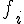743 394 245 190 144 127 115 104 90 81 1182

### Chao1 下界估計量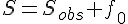``````fau <- cbind(1:10, c(743, 394, 245, 190, 144, 127, 115, 104, 90, 81))
par(lwd = 2, pch = 19, cex = 1.3)
if (Sys.info()["sysname"] == "Darwin") par(family = "STKaiti")
plot(fau, type = "b", main = "紅樓夢字彙頻率", xlab = "字彙出現次數 (i)",
ylab = "字彙種類數 (fi)", xlim = c(0, 10), ylim = c(80, 900))
lines(c(0, 1), c(900, 743), lty = 3)
points(0, 900, pch = 1, lwd = 2)``````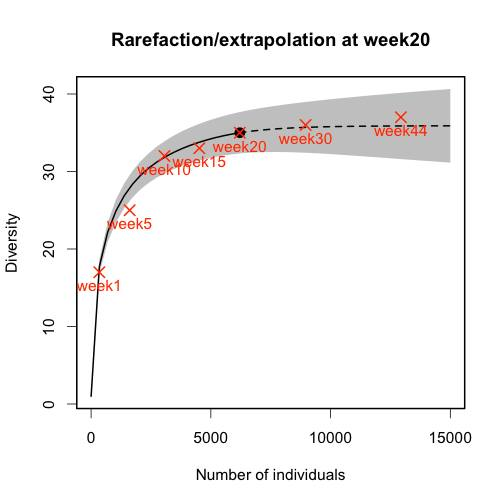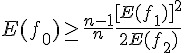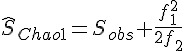### 種類數的累積曲線

``````# library(devtools) install_github('iNEXT','JohnsonHsieh')
library(iNEXT)
encoding = "big5"), row.names = 1)
Sobs <- apply(dat, 2, function(x) sum(x > 0))
n <- apply(dat, 2, function(x) sum(x))

out <- iNEXT(dat\$week20, datatype = "abundance", end = 15000)
par(lwd = 2, pch = 19, cex = 1.3)
plot.iNEXT(out, main = "Rarefaction/extrapolation at week20")
points(n, Sobs, col = 2, pch = 4, cex = 1.5, lwd = 2)
text(n, Sobs, colnames(dat), col = 2, pos = 1, cex = 1)``````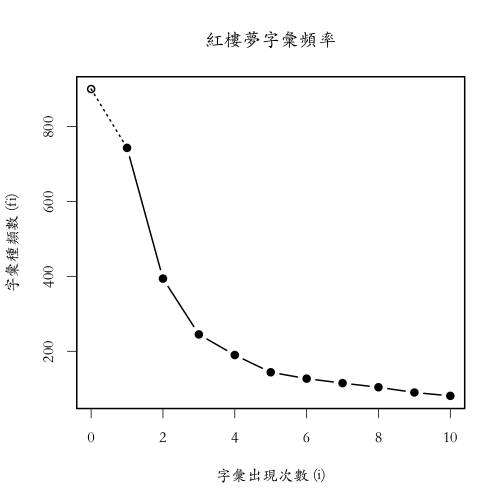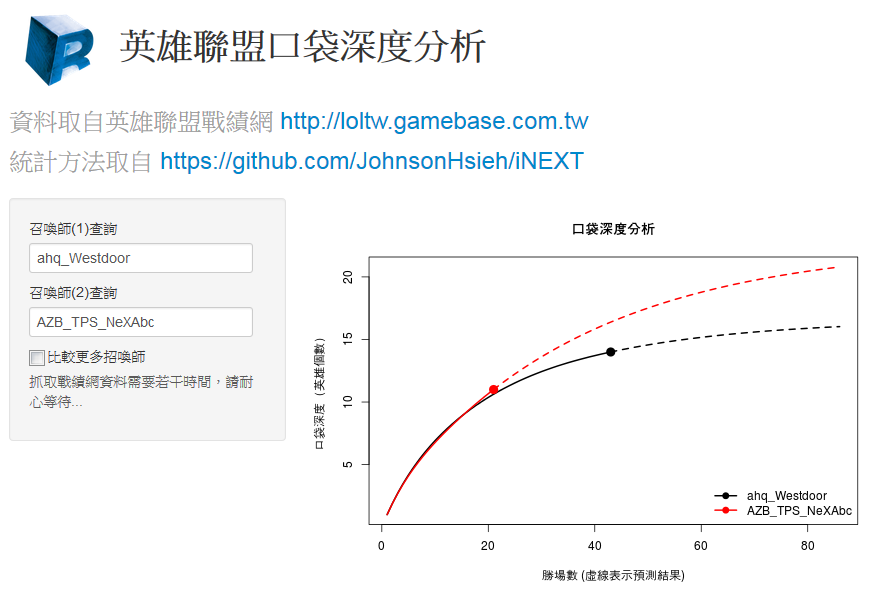LOLChampion

### 參考文獻

• Chao, A. 1984. Nonparametric estimation of the number of classes in a population. Scandinavian Journal of Statistics 11:265-270.
• Colwell, R. K., A. Chao, N. J. Gotelli, S. Y. Lin, C. X. Mao, R. L. Chazdon, and J. T. Longino. 2012. Models and estimators linking individual-based and sample-based rarefaction, extrapolation and comparison of assemblages. Journal of Plant Ecology 5:3-21.
• Sanders, H. L. 1968. Marine benthic diversity: a comparative study. American Naturalist 102:243-282.
• Shen, T. J., A. Chao, and C. F. Lin. 2003. Predicting the number of new species in further taxonomic sampling. Ecology 84:798-804.

### 作者

#### T.C. (Johnson) Hsieh ( <!-- h='&#x67;&#x6d;&#x61;&#x69;&#108;&#46;&#x63;&#x6f;&#x6d;';a='&#64;';n='&#x65;&#x75;&#108;&#x65;&#114;&#x39;&#54;';e=n+a+h; document.write('<a h'+'ref'+'="ma'+'ilto'+':'+e+'">'+e+'<\/'+'a'+'>'); // --> euler96 at gmail dot com)

• 清華統計所博士(2009-2013)，致力於發展沒人懂的統計方法與開發沒人用的統計軟體
• Taiwan R User Group Officer
• Data Science Program 籌備委員
• R 相關著作：
• R套件，CARE1主要作者
• R套件，iNEXT主要作者
• Shiny應用，iNEXT-Online
• 研究領域：Statistics, ecology and genetics